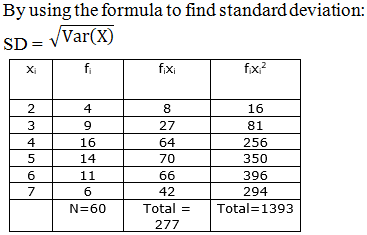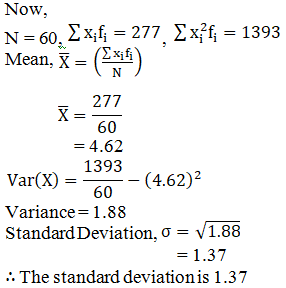# RD Sharma Solutions for Class 11 Chapter 32 - Statistics Exercise 32.5

In this section, we shall deal with variance of a discrete frequency distribution, which are illustrated with examples. Students can use this exercise as a model of reference to improve their conceptual knowledge and understand the different methodology used to solve the problems. Students can refer to RD Sharma Class 11 Maths Solutions, which is one of the best reference guides they can depend upon. Solutions here are developed by the subject experts in a simpler manner to help increase students’ confidence, which plays a major role in their board exams. The links to the solutions of this exercise can be accessed in the RD Sharma Class 11 Maths pdf, which is available in the below-mentioned links.

## Download the pdf of RD Sharma Solutions for Class 11 Maths Exercise 32.5 Chapter 32 – Statistics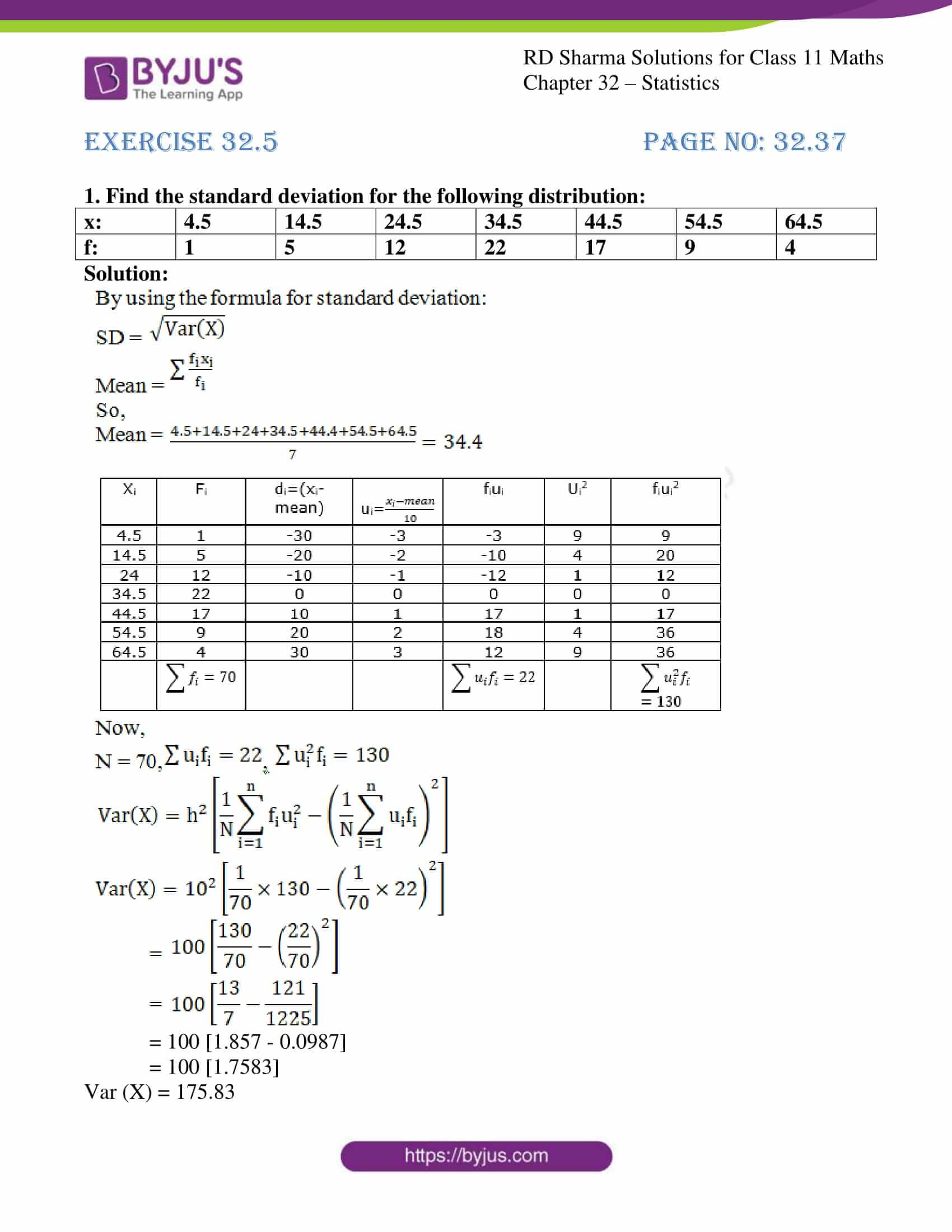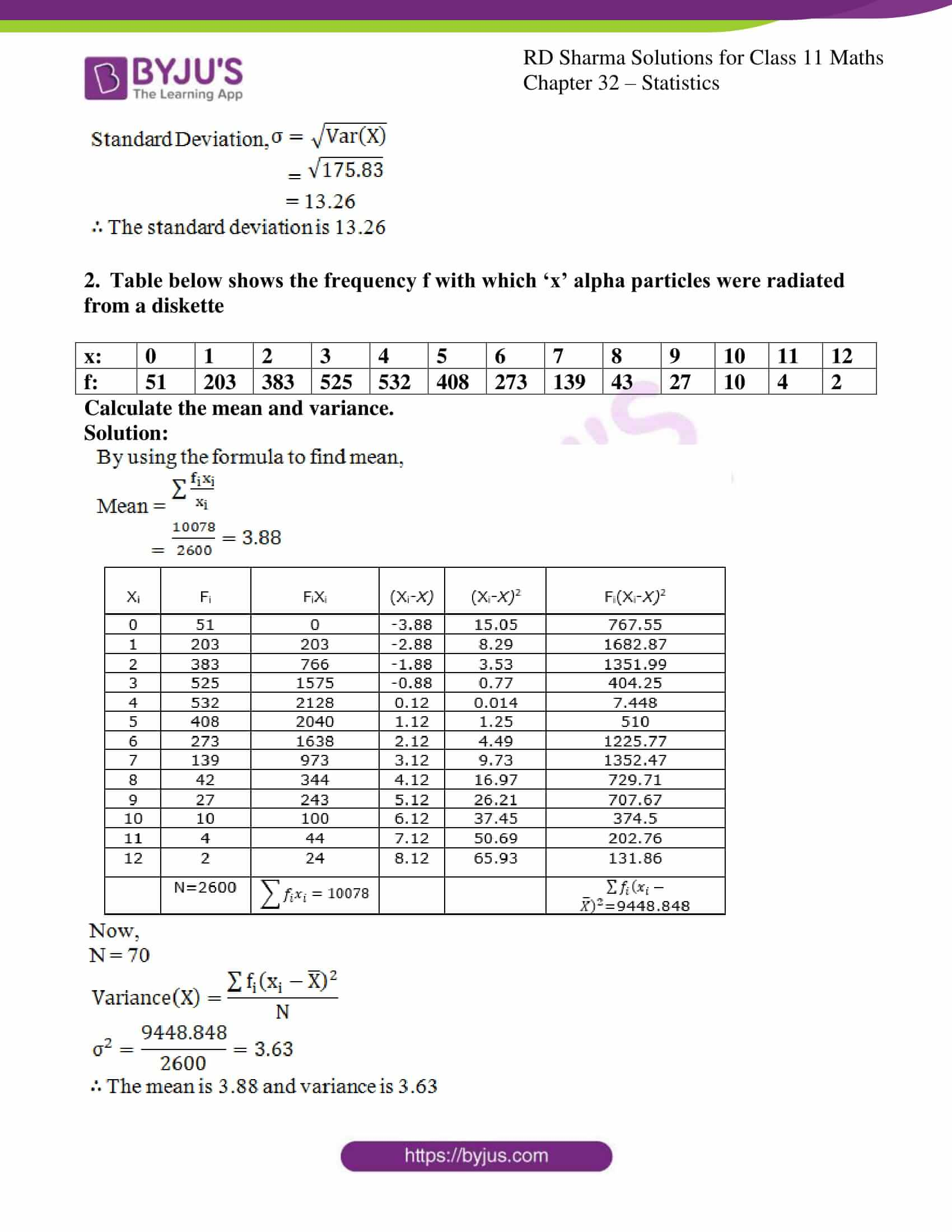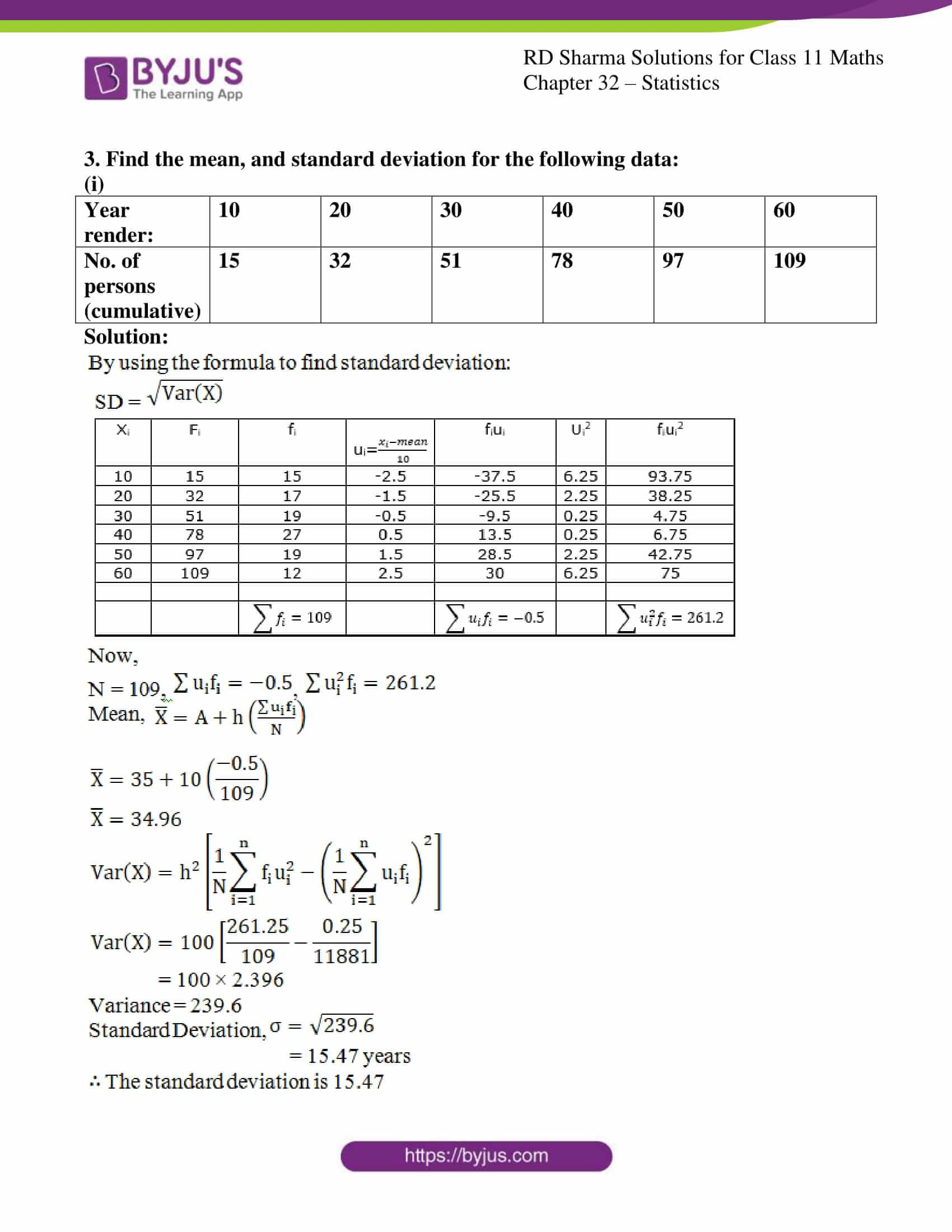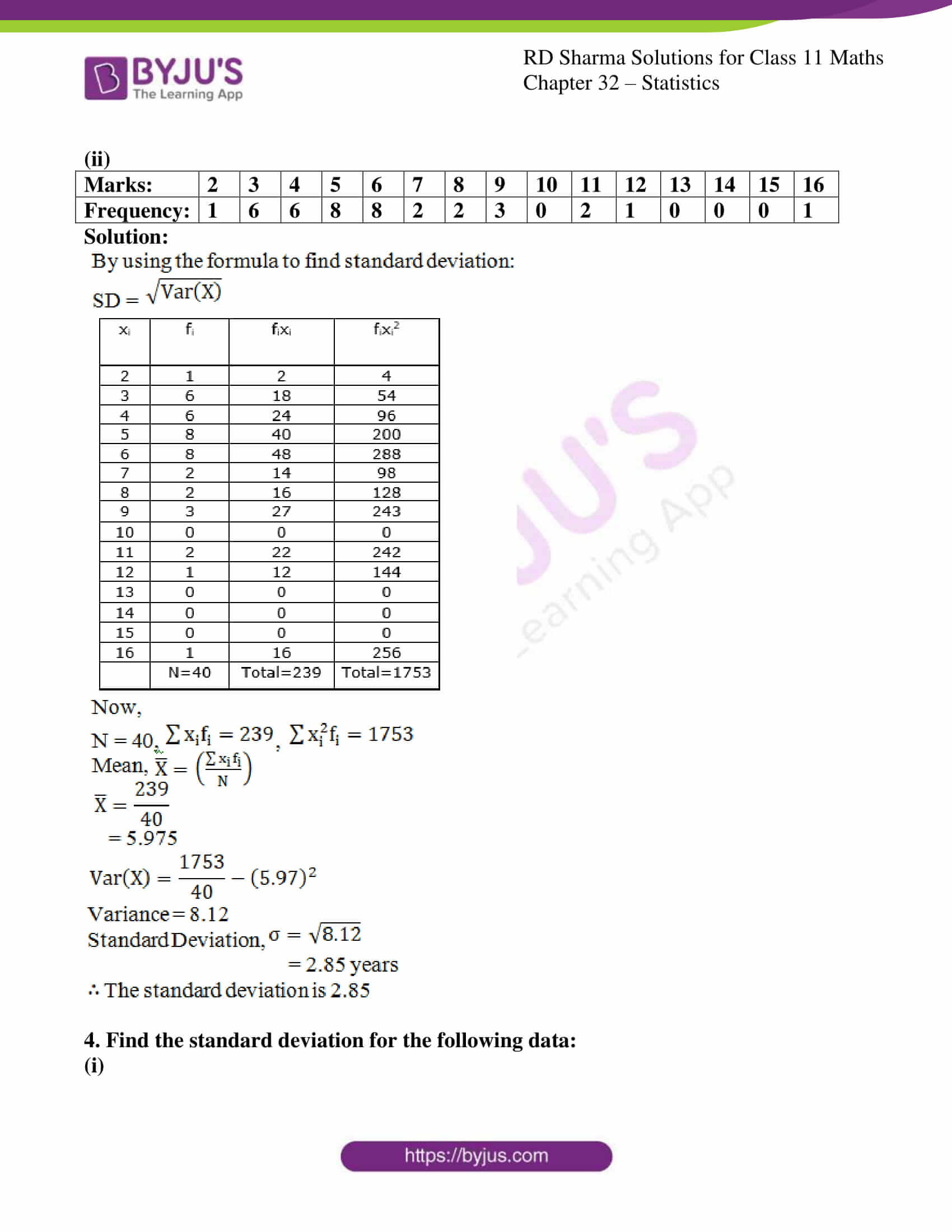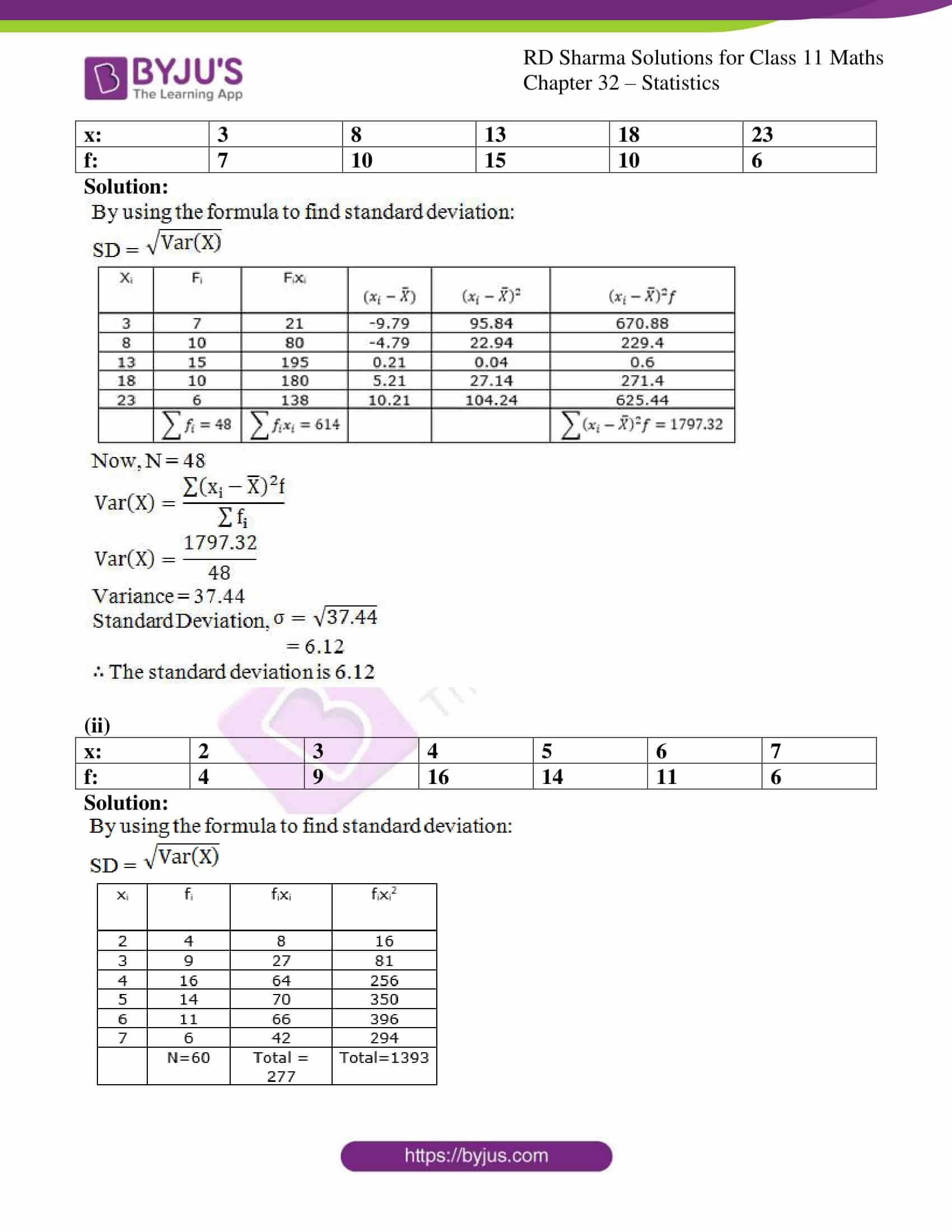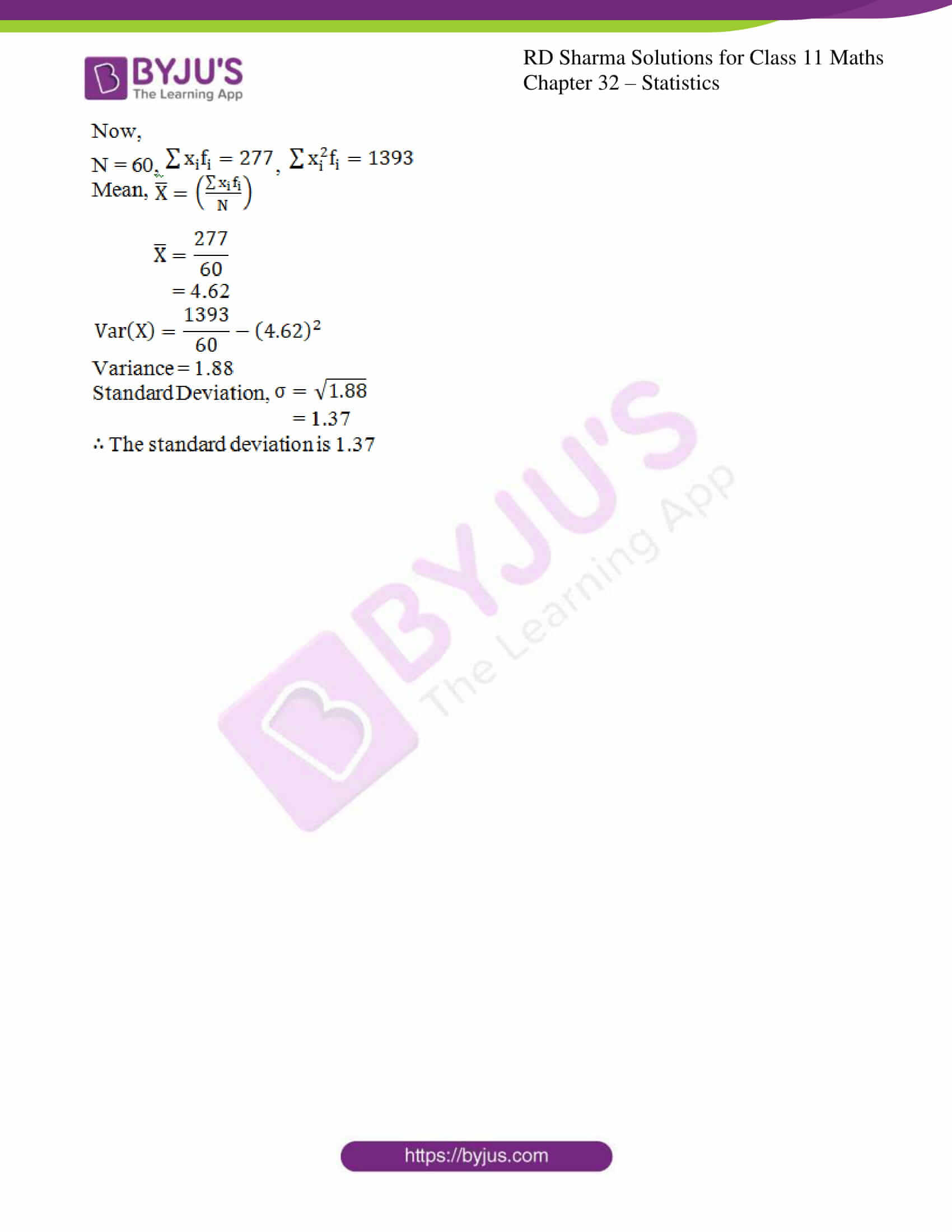### Access answers to RD Sharma Solutions for Class 11 Maths Exercise 32.5 Chapter 32 – Statistics

#### EXERCISE 32.5 PAGE NO: 32.37

1. Find the standard deviation for the following distribution:

 x: 4.5 14.5 24.5 34.5 44.5 54.5 64.5 f: 1 5 12 22 17 9 4

Solution: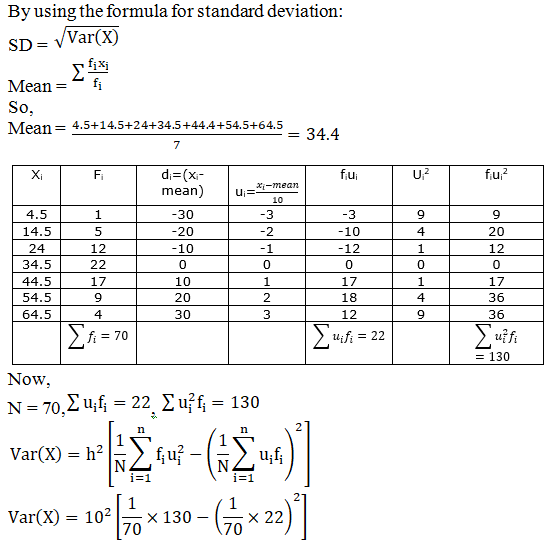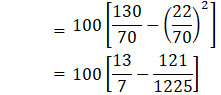= 100 [1.857 – 0.0987]

= 100 [1.7583]

Var (X) = 175.83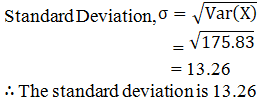2. Table below shows the frequency f with which ‘x’ alpha particles were radiated from a diskette

 x: 0 1 2 3 4 5 6 7 8 9 10 11 12 f: 51 203 383 525 532 408 273 139 43 27 10 4 2

Calculate the mean and variance.

Solution: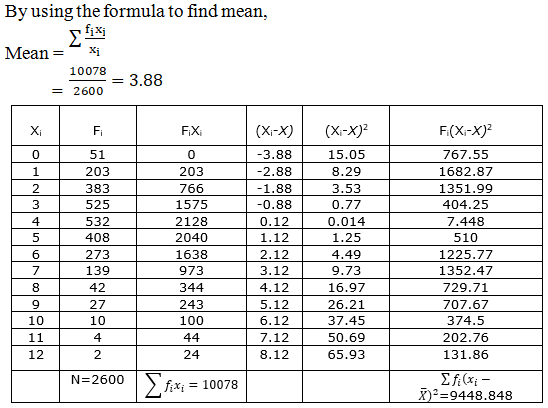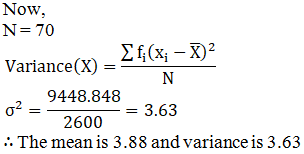3. Find the mean, and standard deviation for the following data:
(i)

 Year render: 10 20 30 40 50 60 No. of persons (cumulative) 15 32 51 78 97 109

Solution: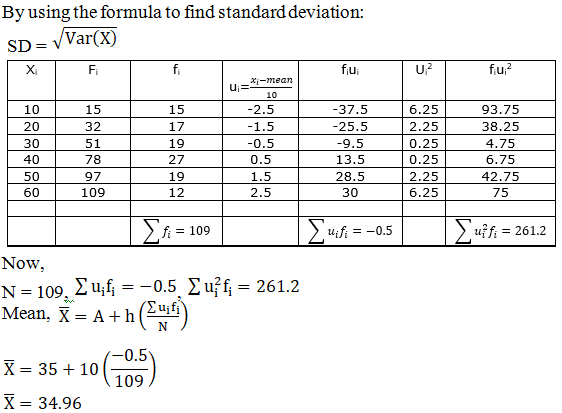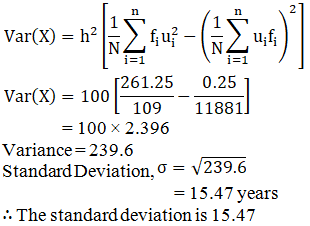(ii)

 Marks: 2 3 4 5 6 7 8 9 10 11 12 13 14 15 16 Frequency: 1 6 6 8 8 2 2 3 0 2 1 0 0 0 1

Solution:4. Find the standard deviation for the following data:

(i)

 x: 3 8 13 18 23 f: 7 10 15 10 6

Solution: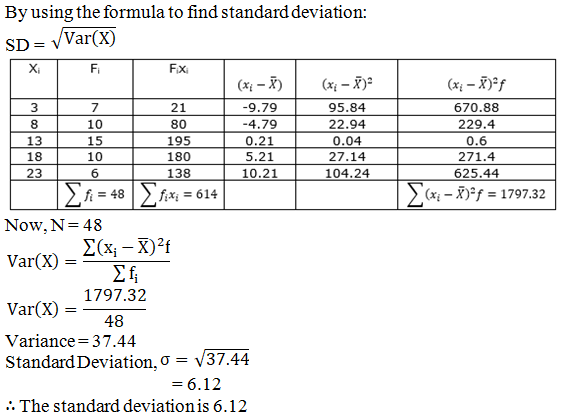(ii)

 x: 2 3 4 5 6 7 f: 4 9 16 14 11 6

Solution: